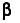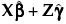Actual by Conditional Predicted Plot Plots actual values versus values predicted by the model, accounting for the random effects. When there are random effects, the Actual by Conditional Predicted Plot appears by default. Actual by Conditional Predicted Plot Conditional Residual Plots Provides residual plots that assess model fit, accounting for the random effects. Conditional Residual Plots Conditional Profiler, Conditional Contour Profiler, Conditional Mixture Profiler, Conditional Surface Profiler Provides profilers to examine the relationship between the response and the model terms, accounting for random effects. Conditional Profilers Variogram Provides a variogram plot that shows the change in covariance as the distance between observations increases. When the Residual structure is selected, you can select the columns to use as temporal or spatial coordinates. Variogram
The Actual by Conditional Predicted plot appears by default. It provides a visual assessment of model fit that accounts for variation due to random effects. It plots the observed values of Y against the conditional predicted values of Y. These are the predicted values obtained if you select Save Columns > Conditional Prediction Formula.
Denote the linear mixed model by E[Y|γ] = Xβ + Zγ. Hereis the vector of fixed effect coefficients and γ is the vector of random effect coefficients. The conditional predictions are the predictions obtained from the model given by.
Denote the linear mixed model by E[Y|γ] = Xβ + Zγ. Hereis the vector of fixed effect coefficients and γ is the vector of random effect coefficients. The conditional residuals are given as follows:
Shows the conditional residuals plotted against the conditional predicted values of Y. You typically want to see the conditional residual scattered randomly about zero.
Denote the linear mixed model by E[Y|γ] = Xβ + Zγ. Hereis the vector of fixed effect coefficients and γ is the vector of random effect coefficients. The conditional predictions are the predictions obtained from the model given by.
 • Conditional Profiler
 • Conditional Contour Profiler
 • Conditional Mixture Profiler
 • Conditional Surface Profiler
Options that are appropriate for the model that you are fitting are enabled. See Marginal Profiler Plot for Treatment A for an example of a Profiler. See Surface Profiler Showing the Response Surface for MODULUS and Silica = 1.2 for an example of a Surface Profiler. For details about the profiler, see the Profilers book.
The nugget is the vertical jump from the value of 0 at the origin of the variogram to the value of the semivariance at a very small separation distance. A variogram model with a nugget has a discontinuity at the origin. The value of the theoretical curve for distances just above 0 is the nugget.